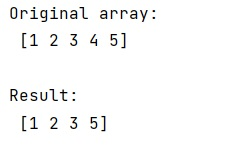# Delete an object from NumPy array without knowing index

Learn, how can we delete an object from a NumPy array without knowing the index in Python? By Pranit Sharma Last updated : March 31, 2023

Suppose that we are given a numpy array that contains some numerical values and we need to delete an element from this numpy array but we do not know its index.

## Deleting an object from NumPy array without knowing index

Since we do not know the index of the values that we want to delete, hence, the first step is to get the index of the values which we want to delete.

To delete an object from NumPy array without knowing the index, we can get the index of the values with the help of argwhere() where we can pass a condition arr == value which will return the index of the value and finally we will delete the value using numpy.delete() method.

Let us understand with the help of an example,

## Python code to delete an object from a NumPy array without knowing the index

```# Import numpy
import numpy as np

# Creating a numpy array
arr = np.array([1,2,3,4,5])

# Display Original array
print("Original array:\n",arr,"\n")

# Getting index of 4
ind = np.argwhere(arr==4)

# Deleting 4
res = np.delete(arr,ind)

# Display result
print("Result:\n",res,"\n")
```

### Output